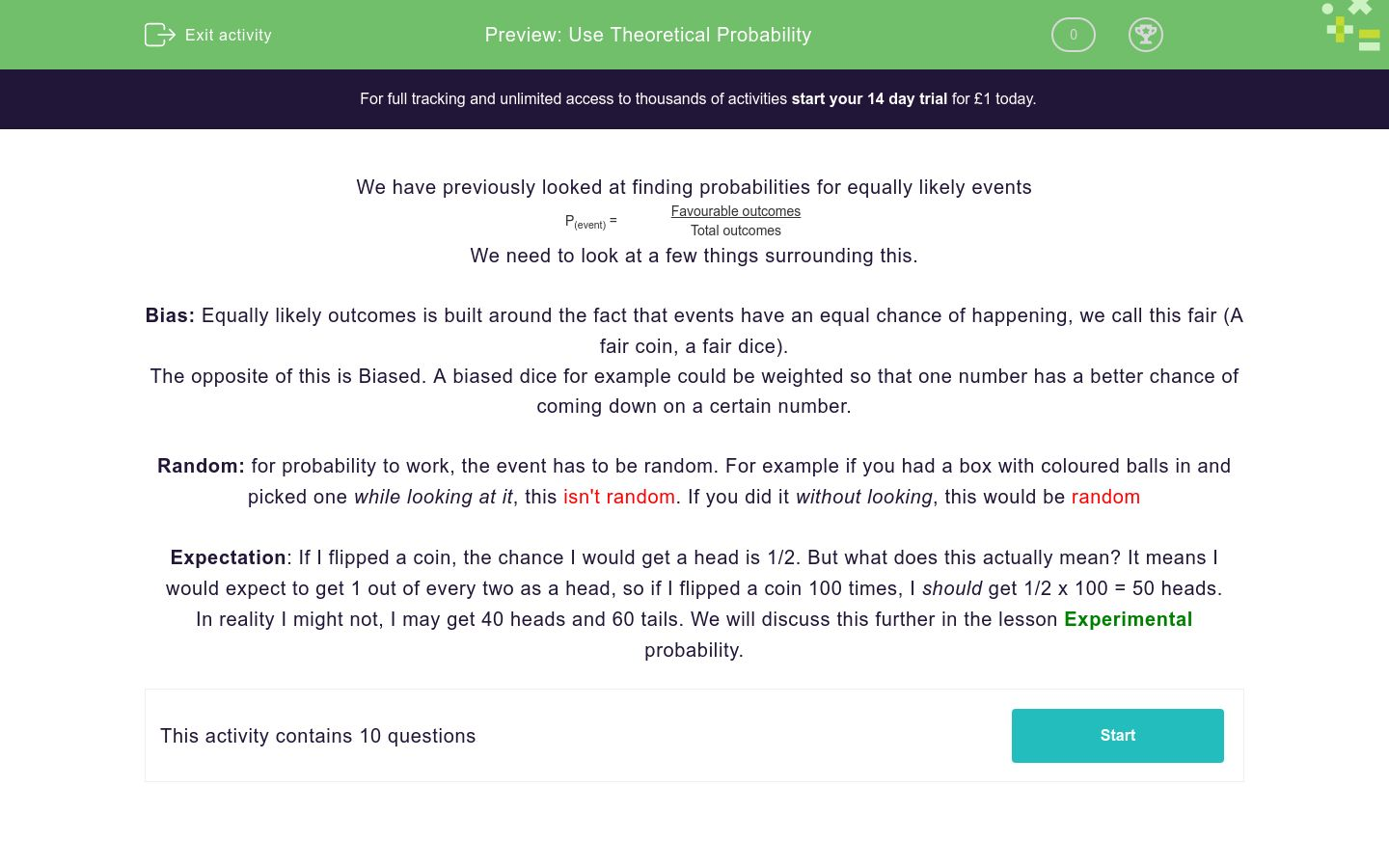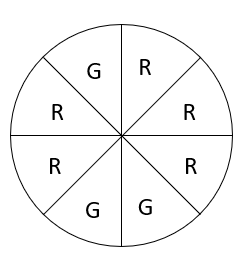# Use Theoretical Probability

In this worksheet, students practise finding theoretical probabilities.Key stage:  KS 4

GCSE Subjects:   Maths

GCSE Boards:   AQA, Eduqas, Pearson Edexcel, OCR

Curriculum topic:   Probability

Curriculum subtopic:   Probability, Basic Probability and Experiments

Difficulty level:### QUESTION 1 of 10

We have previously looked at finding probabilities for equally likely events

P(event) =
 Favourable outcomes Total outcomes

We need to look at a few things surrounding this.

Bias: Equally likely outcomes is built around the fact that events have an equal chance of happening, we call this fair (A fair coin, a fair dice).

The opposite of this is Biased. A biased dice for example could be weighted so that one number has a better chance of coming down on a certain number.

Random: for probability to work, the event has to be random. For example if you had a box with coloured balls in and picked one while looking at it, this isn't random. If you did it without looking, this would be random

Expectation: If I flipped a coin, the chance I would get a head is 1/2. But what does this actually mean? It means I would expect to get 1 out of every two as a head, so if I flipped a coin 100 times, I should get 1/2 x 100 = 50 heads.

In reality I might not, I may get 40 heads and 60 tails. We will discuss this further in the lesson Experimental probability.

A coin had blu-tack stuck to it before it is flipped.

Is this coin fair or biased?

Fair

Biased

Is this spinner fair?Yes

No

For each of these situations, select if they are random or not random.

I throw a fair dice 60 times. How many times should I get the number 3?

I throw a fair dice 60 times. How many times will I get the number 3?

10

30

We can't tell

I throw a fair coin 200 times. How many times should I get a head?

I throw a fair coin 200 times. How many times should it land (and stay) on it's edge?

Match the situation to the number

## Column B

How many square numbers if I roll a dice 300 times...
125
I throw a coin 250 times, how many heads should I ...
350
A bag contains 7 red and 3 blue balls, If I take a...
100

For each of these situations, select if it is fair or biased.

I pick a card out of a deck of cards at random, record the suit and replace it.

If I do this 1000 times, how many Hearts should I get?

• Question 1

A coin had blu-tack stuck to it before it is flipped.

Is this coin fair or biased?

Biased
EDDIE SAYS
If we stick blu-tack to a coin, it is heavier on one side so that side is more likely to land downwards. Because the outcomes are not equal. The coin is biased.
• Question 2

Is this spinner fair?No
EDDIE SAYS
There are more red sections that green sections, this means the outcomes are not equally likely and the spinner is unfair.
• Question 3

For each of these situations, select if they are random or not random.

EDDIE SAYS
The key to this is the question 'is the thing I pick influenced by something' If you can't influence the outcome, the event is random.
• Question 4

I throw a fair dice 60 times. How many times should I get the number 3?

10
EDDIE SAYS
There is one way of getting a 3 and six outcomes in total. The probability of getting a 3 is 1/6 If I throw a dice 60 times, I should get 1/6 x 60 number 3s
• Question 5

I throw a fair dice 60 times. How many times will I get the number 3?

We can't tell
EDDIE SAYS
Did you notice the word change? We can say how many we should get, but we can't say how many we will get.
• Question 6

I throw a fair coin 200 times. How many times should I get a head?

10
EDDIE SAYS
There is one way of head and two outcomes in total. The probability of getting a head is 1/2 If I throw a coin 200 times, I should get 1/2 x 200 heads
• Question 7

I throw a fair coin 200 times. How many times should it land (and stay) on it's edge?

0
EDDIE SAYS
Has anyone ever actually seen this happen? This is so close to impossible we can say it is impossible and we shouldn't get any.
• Question 8

Match the situation to the number

## Column B

How many square numbers if I roll...
100
I throw a coin 250 times, how man...
125
A bag contains 7 red and 3 blue b...
350
EDDIE SAYS
This is all about using a process. 1) Find the probability of the event happening 2) Multiply this by the number of trials (the amount of times you do something)
• Question 9

For each of these situations, select if it is fair or biased.

EDDIE SAYS
The key question to ask here is 'is every outcomes equally likely?' If it isn't, the event is biased.
• Question 10

I pick a card out of a deck of cards at random, record the suit and replace it.

If I do this 1000 times, how many Hearts should I get?

250
EDDIE SAYS
There are 13 hearts in a deck of cards out of 52 cards. This gives us a probability of 13/52. This is hard to deal with so we cancel down and get a probability of 1/4 This means 1/4 of the cards I pull out should be a heart.
---- OR ----

Sign up for a £1 trial so you can track and measure your child's progress on this activity.

### What is EdPlace?

We're your National Curriculum aligned online education content provider helping each child succeed in English, maths and science from year 1 to GCSE. With an EdPlace account you’ll be able to track and measure progress, helping each child achieve their best. We build confidence and attainment by personalising each child’s learning at a level that suits them.

Get started